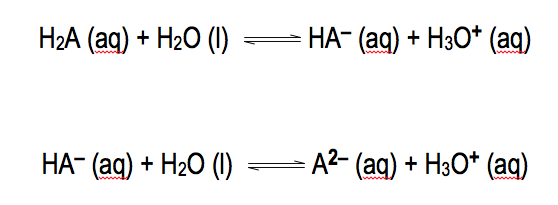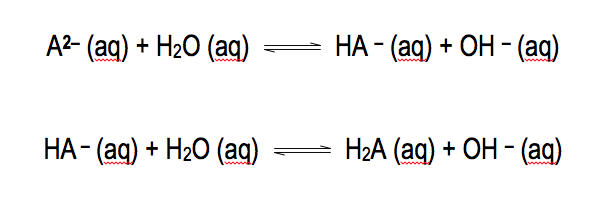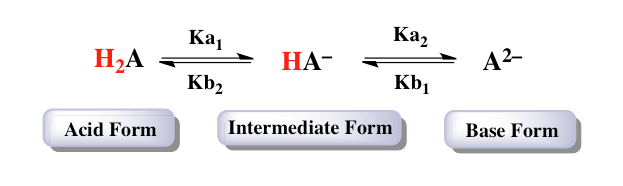Clutch Prep is now a part of Pearson
Ch.9 - Polyprotic Acid-Base EquilibriaWorksheetSee all chapters

# Diprotic Acids and Bases

See all sections
Sections
Diprotic Acids and Bases
Polyprotic Acids and Bases
Diprotic Buffers
Polyprotic Buffers
Principal Species
Isoelectric and Isoionic pH

Diprotic species involve the movement of two acidic hydrogens.

###### Diprotic Acids and Bases

Concept #1: Diprotic acids can donate two acidic hydrogens and as a result possess two Ka values.Concept #2: Diprotic bases can accept two acidic hydrogens and as a result possess two Kb values.Concept #3: With the presence of different forms it becomes essential to know which Ka or Kb value to use.###### Diprotic Acids and Bases Calculations

Example #1: Sulfurous acid, H2SO3, represents a diprotic acid with a Ka1 = 1.6 x 10-2 and Ka2 = 4.6 x 10-5. Calculate the pH and concentrations of H2SO3, HSO3 and SO32– when given 0.200 M H2SO3

Example #2: Determine the pH of 0.080 M Na2S. Hydrosulfuric acid, H2S, contains Ka1 = 1.0 x 10-7 and Ka2 = 9.1 x 10-8.

Example #3: If Ka1 = 4.46 x 10-7 and Ka2 = 4.69 x 10-11 for H2CO3 what is the pH for a 0.15 M solution of NaHCO3

Practice: An unknown diprotic acid has an initial concentration of 0.025 M. What is the pH of the solution if pka1 is  3.25 and pKa2 is 6.82?